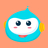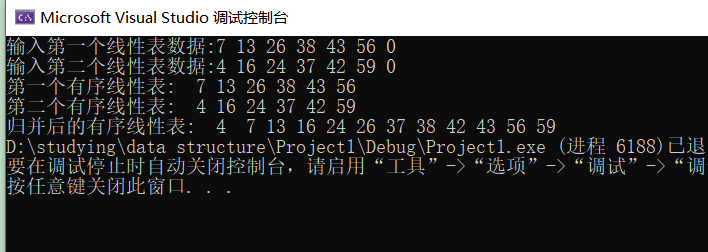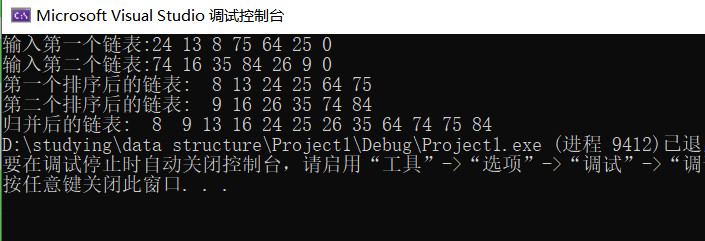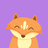• 查找：对于长度为n线性表最坏查找次数
千次阅读
2019-12-20 22:04:21

查找：对于长度为n的线性表最坏查找次数
顺序查找 ----------------------------------n
查找最大项和最小项--------------------n-1
二分查找法----------------------------------log2^n
冒泡排序法----------------------------------n(n-1)/2
快速排序法---------------------------------n(n-1)/2
简单插入排序-------------------------------n(n-1)/2
希尔排序法-----------------------------------n^1.5
简单选择排序-------------------------------n(n-1)/2
堆排序------------------------------------------nlog2^n数据结构
更多相关内容
• 满意答案shulilv2013.11.29采纳率：43%等级：11已帮助：11086人#include int input(int n){printf("请输入这%d个数: \n",n);int i, a[n];for(i=0;i{scanf("%d",&a[i]);if(a[i]}return 1;}bool bsearch(int left,...

满意答案shulilv

2013.11.29采纳率：43%    等级：11

已帮助：11086人

#include

int input(int n)

{

printf("请输入这%d个数: \n",n);

int i, a[n];

for(i=0;i

{

scanf("%d",&a[i]);

if(a[i]

}

return 1;

}

bool bsearch(int left, int right, int k)

{

if(!input()) { printf("输入错误，请重新运行程序。"); return false;}

else

if(left<=right)

{

int mid;

left = 0; right = n; mid = (left+right)/2;

if(k==a[mid]) { printf("a[%d]=%d",mid,k); return true;}

else if(k

展开全文• 将这两个有序线性表归并一个有序线性表；输出归并后的有序线性表。 2.从键盘实现数据输入与输出的格式自拟；要求完成两个同样功能的程序，一个程序采用顺序存储结构，另一个程序采用链表实现线性表的存储。其中...

一、实验内容及要求：

1.从键盘输入数据，建立两个有序线性表(每个线性表的输入数据按由小到大次序输入来建立线性表，不必考虑排序算法)；输出建好的这两个有序线性表；将这两个有序线性表归并为一个有序线性表；输出归并后的有序线性表。

2.从键盘实现数据输入与输出的格式自拟；要求完成两个同样功能的程序，一个程序采用顺序存储结构，另一个程序采用链表实现线性表的存储。其中链表实现时，要求利用两个升序链表的结点实现归并，即归并时不能新建结点，归并后原来两个升序链表的存储空间不在存在。

二、主要说明

1.数据结构设计简要描述：

顺序存储结构使用结构体，结构体中带有数组以及数组的最大存储空间。

链式存储结构同样使用结构体定义结点，用带附加头结点单向链表，每个结点包括整型或浮型类型的数据域和一个指针域。

2.算法设计简要描述：

顺序与链式两种存储均为依次互相比较两线性表中值的大小。顺序存储是新申请存储空间将数据依次存入静态数组中，链式存储是利用原有空间将结点按照数据大小进行重新连接。

第一个程序是通过动态分配内存，初始化两个线性表，然后通过两表相同索引之间值的比较，将其重新赋给新建立的第三个表从而实现两表的合并；

第二个程序是通过建立表头，设置循环分别建立两个链表，然后通过两表中data数值的比较，使第三个表等于第一个表，排序后全部插入第一个表，最后释放第二个表，从而实现量表的合并。

3.输入/输出设计简要描述：

第一个程序从键盘以从小到大的顺序输入以空格(或CR或TAB)分隔的若干不等于0的整数，直到输入0时停止输入，按整数输入次序建立结点并顺序连接结点。

第二个程序从键盘乱序输入以空格(或CR或TAB)分隔的若干不等于0的整数，直到输入0时停止输入，按排序后整数输入次序建立结点并顺序连接结点。

4.编程语言说明：

编程平台：Visual Stdio 2019。编程语言：c语言。

5.主要函数说明：

第一个程序：首先通过InitList函数为线性表动态分配空间，然后通过Input为线性表赋值，初始化好后通过MergeList函数合并两线性表，最后通过Output函数输出线性表。

第二个程序：首先通过CreateList函数在动态创建表头的同时依次指向下一个节点并赋值，再用函数SortList给输入的数排序，然后通过MergeList合并两线性表，最后用OutputList输出线性表。

三、源程序代码

程序一：

运行示例：程序源码：

#include<stdio.h>
#include<stdlib.h>
#define LIST_INIT_SIZE 100
typedef struct {
int *elem;
int length;
int listsize;
}SqList;
void InitList(SqList &L) {
L.elem = (int *)malloc(LIST_INIT_SIZE * sizeof(int));
if (!L.elem) exit(1);
L.length = 0;
L.listsize = LIST_INIT_SIZE;
}
void Input(SqList& L) {
int x;
while (1) {
scanf_s("%d", &x);
if (x == 0 || L.length >= LIST_INIT_SIZE) break;
L.elem[L.length++] = x;
}
}
void Output(SqList &L) {
for (int i = 0; i < L.length; i++) {
printf("%3d", L.elem[i]);
}
}
void MergeList(SqList &La, SqList &Lb, SqList &Lc) {
int i = 0, j = 0, k = 0;
Lc.length = La.length + Lb.length;
while (i < La.length && j < Lb.length) {
if (La.elem[i] < Lb.elem[j]) {
Lc.elem[k] = La.elem[i];
i++;
}
else {
Lc.elem[k] = Lb.elem[j];
j++;
}
k++;
}
while (k < Lc.length) {
if (i == La.length) {
Lc.elem[k] = Lb.elem[j];
j++;
}
else {
Lc.elem[k] = La.elem[i];
i++;
}
k++;
}
}

int main()
{
SqList a, b, c;
InitList(a);
InitList(b);
InitList(c);
printf("输入第一个线性表数据:");
Input(a);
printf("输入第二个线性表数据:");
Input(b);
printf("第一个有序线性表:");
Output(a);
printf("\n");
printf("第二个有序线性表:");
Output(b);
printf("\n");
MergeList(a, b, c);
printf("归并后的有序线性表:");
Output(c);
}

程序二：

运行示例：程序源码：

#include<stdio.h>
#include<stdlib.h>
#define LIST_INIT_SIZE 100
typedef struct LNode {
int data;
struct LNode* next;
int e;
scanf_s("%d", &e);
while (e) {
p = (LinkList )malloc(sizeof(LNode) * LIST_INIT_SIZE);
p->data = e;
tail->next = p;
tail = p;
scanf_s("%d", &e);
}
tail->next = NULL;
}
while (p) {
printf("%3d", p->data);
p = p->next;
}
}
int temp;
for(p=L;p!=NULL;p=p->next)
for (q = p->next; q != NULL; q = q->next)
{
if (p->data >q->data) {
temp = p->data;
p->data = q->data;
q->data = temp;
}
}
}
pa = La->next;
pb = Lb->next;
pc = La;
free(Lb);
while(pa && pb)
if (pa->data <= pb->data) {
pc->next = pa;
pc = pa;
pa = pa->next;
}
else {
pc->next = pb;
pc = pb;
pb = pb->next;
}
if (pa) pc->next = pa;
else pc->next = pb;
}
int main()
{
printf("输入第一个链表:");
La = CreatList();
SortList(La);
printf("输入第二个链表:");
Lb = CreatList();
SortList(Lb);
printf("第一个排序后的链表:");
OutputList(La);
printf("\n");
printf("第二个排序后的链表:");
OutputList(Lb);
MergeList(La, Lb);
printf("\n");
printf("归并后的链表:");
OutputList(La);
}

展开全文数据结构 算法 链表
• //序号i处放入x L->length++; return 1; } /*归并 增序*/ void merge(sqlist A, sqlist B, sqlist *C) { int m =0, n=0; while (m < A.length && n if(A.data[m] { insert(C,C->length+1,A.data[m]); m++; } ...

满意答案bufulaq

2014.01.27采纳率：53%    等级：11

已帮助：10111人

#include

#include

#include

typedef int ElemType;

#define INITSIZE 100

typedef struct

{

ElemType *data;

int length;

int listsize;

}sqlist;

/*初始化*/

void initlist(sqlist *L,int n)

{

int i;

L->data =(ElemType *)malloc(sizeof(ElemType)*INITSIZE);

L->length=0;

for(i=1;i<=n;i++)

{

scanf("%d",&L->data[i-1]);

L->length++;

}

L->listsize=INITSIZE;

}

/*插入元素*/

int insert(sqlist *L,int i,ElemType x)

{

int j;

if(i<1||i>L->length+1) return 0;

if(L->length==L->listsize) //存储空间不够，增加一个

{

L->data=(ElemType *)realloc(L->data,(L->listsize+1)*sizeof(ElemType));

L->listsize++; //重置存储空间长度

}

for(j=L->length;j>=i;j--)

L->data[j]=L->data[j-1]; //将序号为i的结点及之后的结点后移

L->data[i-1]=x; //在序号为i处放入x

L->length++;

return 1;

}

/*归并 增序*/

void merge(sqlist A, sqlist B, sqlist *C)

{

int m =0, n=0;

while (m < A.length && n

if(A.data[m]

{

insert(C,C->length+1,A.data[m]);

m++;

}

else //将B的data[n]插入C尾部

{

insert(C,C->length+1,B.data[n]);

n++;

}

while(m

{

insert(C,C->length+1,A.data[m]);

m++;

}

while(n

{

insert(C,C->length+1,B.data[n]);

n++;

}

}

/*归并 逆序*/

void merge1(sqlist A, sqlist B, sqlist *C)

{

int m =0, n=0;

while (m < A.length && n

if(A.data[m]

{

insert(C,1,A.data[m]);

m++;

}

else //将B的data[n]插入C头部

{

insert(C,1,B.data[n]);

n++;

}

while(m

{

insert(C,1,A.data[m]);

m++;

}

while(n

{

insert(C,1,B.data[n]);

n++;

}

}

/*输出*/

void list(sqlist *L)

{

int i;

for(i=0;ilength;i++)

printf("%d ",L->data[i]);

printf("\n");

}

/*主函数*/

void main()

{

int m=0,n=0;

sqlist A,B,C;

printf("please input the number of A elemtype M is:");

scanf("%d",&m);

initlist(&A,m);

printf("please input the number of B elemtype N is:");

scanf("%d",&n);

initlist(&B,n);

printf("merge A and B is C:\n");

C.data =(ElemType *)malloc(sizeof(ElemType)*INITSIZE);

C.length=0;

C.listsize=INITSIZE;

merge1(A,B,&C);

list(&C);

}

以上是在VC6.0上运行通过的。

//运行结果:

please input the number of A elemtype M is: 3

3 5 6

please input the number of B elemtype N is: 5

1 4 6 8 9

merge A and B is C:

9 8 6 6 5 4 3 1

02分享举报

展开全文• 已知线性表 LA 和 LB 中的数据元素按值非递减有序排列，现要求将 LA 和 LB 归并一个新的线性表 LC， 且 LC 中的数据元素仍然按值非递减有序排列。例如，设LA=(3,5,8,11) ,LB=(2,6,8,9,11,15,20) 则 LC=(2,3,6,6,8...
•C++ 链表 顺序表 数据结构
• ## 有序线性表的有序合并 （c语言）

千次阅读 多人点赞 2018-09-22 14:21:52
已知线性表 LA 和 LB 中的数据元素按值非递减有序排列，现要求将 LA 和 LB 归并一个新的线性表 LC， 且 LC 中的数据元素仍然按值非递减有序排列。例如，设LA=(3,5,8,11) ,LB=(2,6,8,9,11,15,20) 则 LC=(2,3,6,6,8,...
• 有序线性表查找平均长度 其中n为查找表中元素个数 Pi查找第i个元素的概率,通常假设每个元素查找概率相同，Pi=1/n Ci是找到第i个元素的比较次数。 举例： 长度10的表，采用顺序查找法，平均查找长度...
• 已知线性表 LA 和 LB 中的数据元素按值非递减有序排列，现要求将 LA 和 LB 归并一个新的线性表 LC， 且 LC 中的数据元素仍然按值非递减有序排列。例如，设LA=(3,5,8,11) ,LB=(2,6,8,9,11,15,20) 则 LC=(2,3,6,6,8...
• 由两个递增有序线性表A、B归并新的递减有序线性表C 数据结构题目 严蔚敏版数据结构教材题集2.24 假设有两个按元素值递增有序排列的线性表A和B，均以单链表作存储结构，请编写算法将A表和B表归并成一个按元素值递减...数据结构 c++ 链表
• Description已知线性表 LA 和 LB 中的数据元素按值非递减有序排列，现要求将 LA 和 LB 归并一个新的线性表 LC， 且 LC 中的数据元素仍然按值非递减有序排列。例如，设LA=(3,5,8,11) ,LB=(2,6,8,9,11,15,20) 则LC=...
• (5.0分)【填空题】假设在有序线性表a上进行折半查找,则比较一次查找成功的结点数1;比较两次查找成功的结点数 ( ) ;比较四次查找成功的结点数 ( ) 。【判断题】一个连通图的生成树是一个...
• printf("当前线性表分别：\n"); for(int i=0;i printf("%d ",a.data[i]); } printf("\n"); for(int i=0;i printf("%d ",b.data[i]); } printf("\n"); merge(s,a,b); printf("合并之后：\n"); for(int k=0;k ...c语言 数据结构
• 已知线性表 LA 和 LB 中的数据元素按值非递减有序排列，现要求将 LA 和 LB 归并一个新的线性表 LC， 且 LC 中的数据元素仍然按值非递减有序排列。例如，设LA=(3,5,8,11) ,LB=(2,6,8,9,11,15,20) 则 LC=(2,3,6,6,8...
• 有一个存放整数的长度m+n线性表L，其前m个元素单调递增，后n个元素也单调递增。设计一个算法，使得整个线性表的元素单调递增。要求：使用链式存储实现。有问必答 c语言
• 解题思路:1:分别有三个数组li1,li2,li3;2:chuang1,chuang2,分别是li1,li2的长度；3：g1,g2分别指向li1,li2;4:做比较，当li1[g1]<=li2[g2]时，li3[i]保存li1...调用函数mo,将的字符串的末尾数字输入li3.最后输出...
• 数据结构2.2线性表的顺序表示和实现 严蔚敏版 已知线性表 LA 和 LB 中的数据元素按值非递减有序排列，现要求将 LA 和 LB 归并一个新的线性表 LC， 且 LC 中的数据元素仍然按值非递减有序排列。例如，设LA=(3,5,8,...数据结构 合并 数据
• 有序线性表的插入与删除#include #include # define LIST_INIT_SIZE 100 # define LISTINCREMENT 10 # define ElemType int # define OVERFLOW -1 # define ERROR -1 using namespace std; typedef struct {数据结构
• #include #include using namespace std; #define SIZE_A 2 #define SIZE_B 3 #define SIZE_AB SIZE_A+SIZE_B //2+3=5 int main(){ void showVector(vector *vector_p);//函数提前声明 ... vector list_b
• HNCU1324 算法2-2 有序线性表的有序合并 线性表
• 问题描述：线性表(a1, a2, ..., an)中元素递增有序且按... 分析：顺序存储的线性表递增有序，结合题目要求，故可以使用折半查找法（时间复杂度log2n（以2底，n的对数））。void serachExchangeInsert(int *a...数据结构 二分查找
• 长度为n线性表，最坏情况下查找或比较次数： 类型 次数 顺序查找 n 查找最大项或最小项 n-1 二分法查找 log2n 冒泡排序 n(n-1)/2 快速排序 n(n-1)/2 简单插入排序 n(n-1)/2 堆排序 nlog2n ...
• 题目 1674: 算法2-2：有序线性表的有序合并 题目描述 已知线性表 LA 和 LB 中的数据元素按值非递减有序排列，现要求将 LA 和 LB 归并一个新的线性表 LC， 且 LC 中的数据元素仍然按值非递减有序排列。例如，设LA=...数据结构
• 3.有序表 1.顺序结构 # include # include # include # include using namespace std ; # define MAXSIZE 100 typedef int ElemType ; typedef struct { ElemType * elem ; //存储...
• 将俩有序顺序表合并一个新的有序顺序表，并返回结果 输入： 3 3 1 2 3 4 5 6 输出 1 2 3 4 5 6 输入： 3 3 3 3 4 4 5 5 输出： 3 4 5 思路： 简单比较两个数组大小， 由于有序， 1.如果结果数组当前存储和a...数据结构 算法
• ## 线性表--有序表

千次阅读 2018-08-09 22:40:23
所谓有序表，是指这样的线性表，其中所有元素以递增或递减方式有序排列。为了简单，假设有序表元素是以递增方式排列。从中看到，有序表和线性表中元素之间的逻辑关系相同，其区别是运算实现的不同。 1.有序表基本......